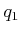#### Pseudometrics

Many planning algorithms use functions that behave somewhat like a distance function but may fail to satisfy all of the metric axioms. If such distance functions are used, they will be referred to as pseudometrics. One general principle that can be used to derive pseudometrics is to define the distance to be the optimal cost-to-go for some criterion (recall discrete cost-to-go functions from Section 2.3). This will become more important when differential constraints are considered in Chapter 14.

In the continuous setting, the cost could correspond to the distance traveled by a robot or even the amount of energy consumed. Sometimes, the resulting pseudometric is not symmetric. For example, it requires less energy for a car to travel downhill as opposed to uphill. Alternatively, suppose that a car is only capable of driving forward. It might travel a short distance to go forward fromto some, but it might have to travel a longer distance to reachfrombecause it cannot drive in reverse. These issues arise for the Dubins car, which is covered in Sections 13.1.2 and 15.3.1.

An important example of a pseudometric from robotics is a potential function, which is an important part of the randomized potential field method, which is discussed in Section 5.4.3. The idea is to make a scalar function that estimates the distance to the goal; however, there may be additional terms that attempt to repel the robot away from obstacles. This generally causes local minima to appear in the distance function, which may cause potential functions to violate the triangle inequality.

Steven M LaValle 2020-08-14